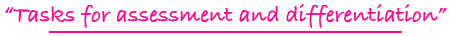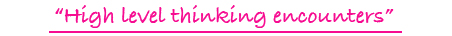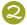•••Tiling Task Card Packets include 20 unique task cards on card stock,  3 backdrop cards , tracking sheets, suggestions and answer sheets.  These are contained in a printed manila envelope for convenient storing.  A set of number tiles 0 - 9 is  needed for each task card

4 - 8

MULTIPLE/FACTOR TILES DIGITAL

finding multiples, including least common multiple, and factors, including greatest common factor

Challenge Level:DIGITAL FORMAT\$10.00

4 - 8

MULTIPLE/FACTOR TILES

finding multiples, including least common multiple, and factors, including greatest common factor

Challenge Level:PDF FORMAT
(You will receive both color and black and white files for printing.)\$10.00

3 - 6

MULTIPLICATION FACT TILES DIGITAL

using a rectangular array as a representational model to form multiplication fact equations

Challenge Level:DIGITAL FORMAT\$10.00

3 - 6

MULTIPLICATION FACT TILES

using a rectangular array as a representational model to form multiplication fact equations

Challenge Level:PDF FORMAT
(You will receive both color and black and white files for printing.)\$10.00

4 - 7

MULTIPLICATION/DIVISION TILES DIGITAL

completing multiplication and division equations and dealing with factors of numbers and multiples of numbers

Challenge Level:DIGITAL FORMAT\$10.00

4 - 7

MULTIPLICATION/DIVISION TILES

completing multiplication and division equations and dealing with factors of numbers and multiples of numbers

Challenge Level:PDF FORMAT
(You will receive both color and black and white files for printing.)\$10.00

4 - 6

MULTIPLY TO A PRODUCT TILES DIGITAL

forming basic multiplication facts with 2 or 3 factors to a given product

Challenge Level:DIGITAL FORMAT\$10.00

4 - 6

MULTIPLY TO A PRODUCT TILES

forming basic multiplication facts with 2 or 3 factors to a given product

Challenge Level:PDF FORMAT
(You will receive both color and black and white files for printing.)\$10.00

5 - 8

MULTIPLY: THINK & TILE

forming a multiplication problem with a 4-digit number as a factor and a single-digit number as a factor by utilizing hints dealing with math concepts and vocabulary

Challenge Level:\$15.00

5 - 8

MULTIPLY: TRIAL & TILE

forming a multiplication problem where a 3-digit number is multiplied by a 2-digit number

Challenge Level:\$15.00

5 - 8

MULTIPLY: TRIAL & TILE DIGITAL

forming a multiplication problem where a 3-digit number is multiplied by a 2-digit number

Challenge Level:DIGITAL FORMAT\$10.00

5 - 8

MULTIPLY: TRIAL & TILE

forming a multiplication problem where a 3-digit number is multiplied by a 2-digit number

Challenge Level:PDF FORMAT
(You will receive both color and black and white files for printing.)\$10.00

4 - 8

NEGATIVE/POSITIVE INTEGER TILES

adding and subtracting of positive and negative whole numbers

Challenge Level:\$15.00

4 - 8

NEGATIVE/POSITIVE INTEGER TILES DIGITAL

adding and subtracting of positive and negative whole numbers

Challenge Level:DIGITAL FORMAT\$10.00

4 - 8

NEGATIVE/POSITIVE INTEGER TILES

adding and subtracting of positive and negative whole numbers

Challenge Level:PDF FORMAT
(You will receive both color and black and white files for printing.)\$10.00

2 - 5

NUMBER SENSE TILES

forming specified numbers using math vocabulary and concepts

Challenge Level:\$15.00

2 - 5

NUMBER SENSE TILES DIGITAL

forming specified numbers using math vocabulary and concepts

Challenge Level:DIGITAL FORMAT\$10.00

2 - 5

NUMBER SENSE TILES

forming specified numbers using math vocabulary and concepts

Challenge Level:PDF FORMAT
(You will receive both color and black and white files for printing.)\$10.00

3 - 6

NUMBER SEQUENCE TILES DIGITAL

sequencing numbers with patterns of plus, minus, or multiples in ascending and descending order

Challenge Level:DIGITAL FORMAT\$10.00

3 - 6

NUMBER SEQUENCE TILES

sequencing numbers with patterns of plus, minus, or multiples in ascending and descending order

Challenge Level:PDF FORMAT
(You will receive both color and black and white files for printing.)\$10.00